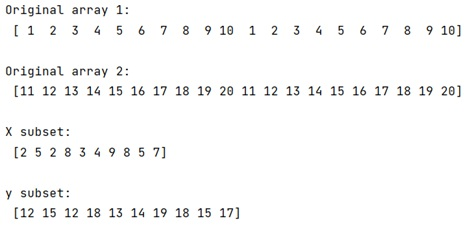# Create Subset of Two NumPy Arrays Using random.sample() with Matching Indices

In this tutorial, we will learn how to create subset of two NumPy arrays using random.sample() with matching indices in Python? By Pranit Sharma Last updated : April 14, 2023

## Overview

Suppose that we are given two numpy arrays x and y, which have lengths 20, we would like to create a random subset of 10 entries of both x and y.

## How to Create Subset of Two NumPy Arrays with Matching Indices?

To create a subset of two NumPy arrays with matching indices, use numpy.random.choice() method which is used to generate a random sample from a given 1-D array. It requires a 1d array with the elements of which the random sample is generated. For a 1D array, we can pass an array created from the indices of either x or y. This will create an array of random indices in the range of length of x.

Once the random indices are created, we can create the subset of x and y by indexing x and y with the random indices respectively.

Let us understand with the help of an example,

## Python Program to Create Subset of Two NumPy Arrays with Matching Indices

```# Import numpy
import numpy as np

# Creating an arrays
x = np.array([1,2,3,4,5,6,7,8,9,10,1,2,3,4,5,6,7,8,9,10])
y = np.array([11,12,13,14,15,16,17,18,19,20,11,12,13,14,15,16,17,18,19,20])

# Display original arrays
print("Original array 1:\n",x,"\n")
print("Original array 2:\n",y,"\n")

# Extracting random indices in range of length of x
idx = np.random.choice(np.arange(len(x)), 10, replace=False)

# Creating subset of x and y
x_s = x[idx]
y_s = y[idx]

# Display result
print("X subset:\n",x_s,"\n")
print("y subset:\n",y_s)
```

### Output